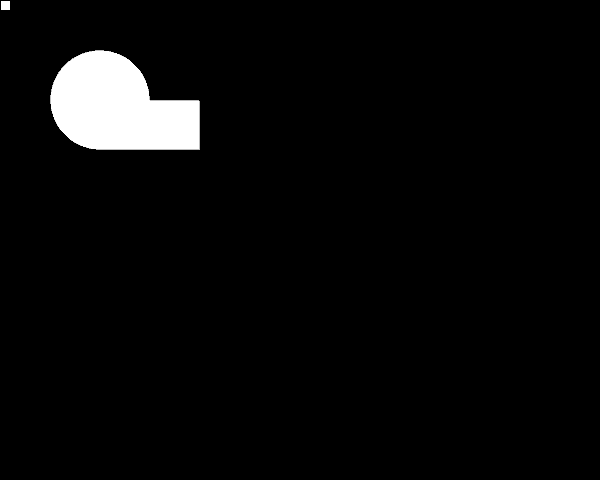Recently there has been some interest in the geomerative library on the processing discourse. Here I describe how to create a custom `RShape` that can detect when another `RShape` is fully contained within the boundary shape. I have done this by extending the `RShape` class to provide the functionality. Here is the `Boundary` class:-

``````java_import 'geomerative.RPoint'
java_import 'geomerative.RShape'

# Shape Boundary Class
class Boundary < RShape
def initialize(shp)
super(shp)
end

def self.create_bounding_shape(shp)
Boundary.new(shp)
end

def inside(shp)
shp.get_points.each { |pt| return false unless contains(pt) }
true
end
end
``````

and here is sketch that demonstrates the functionality:-

``````require 'geomerative'

def settings
size(600, 480, P2D)
end

def setup
sketch_title 'Geomerative Boundary Shape Test'
RG.init(self)
circle = RShape.createCircle(100, 100, 100)
rectangle = RShape.createRectangle(100, 100, 100, 50)
@bounds = Boundary.create_bounding_shape(circle.union(rectangle))
end

def draw
fill 255
stroke 0
@my_rect = RShape.create_rectangle(mouse_x, mouse_y, 10, 10)
bounds.draw
draw_my_rect
end

def draw_my_rect
if bounds.inside(my_rect)
no_stroke
fill 255, 0, 0
else
stroke 0
fill 255
end
my_rect.draw
end
``````

See animated gif created from sketch:-